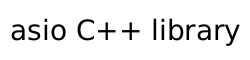require_concept

A customisation point that applies a concept-enforcing property to an object.

constexpr unspecified require_concept = unspecified;

The name require_concept denotes a customization point object. The expression asio::require_concept(E, P) for some subexpressions E and P (with types T = decay_t<decltype(E)> and Prop = decay_t<decltype(P)>) is expression-equivalent to:

• If is_applicable_property_v<T, Prop> && Prop::is_requirable_concept is not a well-formed constant expression with value true, asio::require_concept(E, P) is ill-formed.
• Otherwise, E if the expression Prop::template static_query_v<T> == Prop::value() is a well-formed constant expression with value true.
• Otherwise, (E).require_concept(P) if the expression (E).require_concept(P) is well-formed.
• Otherwise, require_concept(E, P) if the expression require_concept(E, P) is a valid expression with overload resolution performed in a context that does not include the declaration of the require_concept customization point object.
• Otherwise, asio::require_concept(E, P) is ill-formed.